# FORM FOUR PHYSICS STUDY NOTES TOPIC 1: WAVES & TOPIC 2: ELECTROMAGNETISM

1
833TOPIC 1: WAVES

Introduction to Waves
The Concept of Wave
Explain the concept of a wave
A wave is a disturbance that travels through a medium from one location to another location.
Consider
a slinky wave as an example of a wave. When the slinky is stretched
from end to end and is held at rest, it assumes a natural position known
as the equilibrium or rest position.
The
coils of the slinky naturally assume this position, spaced equally far
apart. To introduce a wave into the slinky, the first particle is
displaced or moved from its equilibrium or rest position. The particle
might be moved upwards or downwards, forwards or backwards; but once
moved, it is returned to its original equilibrium or rest position.
The act of moving the first coil of the slinky in a given direction and then returning it to its equilibrium position creates a disturbance
in the slinky. We can then observe this disturbance moving through the
slinky from one end to the other. If the first coil of the slinky is
given a single back-and-forth vibration, then we call the observed
A pulse is a single disturbance moving through a medium from one location to another location.
However,
if the first coil of the slinky is continuously and periodically
vibrated in a back-and-forth manner, we would observe a repeating
disturbance moving within the slinky that endures over some prolonged
period of time. The repeating and periodic disturbance that moves
through a medium from one location to another is referred to as a wave.
A medium is a substance or material that carries the wave.
You have perhaps heard of the phrase news media.
The news media refers to the various institutions (newspaper offices,
television stations, radio stations, etc.) within our society that carry
the news from one location to another. The news moves through the media.
The
wave medium is not the wave and it doesn’t make the wave; it merely
carries or transports the wave from its source to other locations.
In
the case of our slinky wave, the medium through that the wave travels
is the slinky coils. In the case of a water wave in the ocean, the
medium through which the wave travels is the ocean water. In the case of
a sound wave moving from the church choir to the pews, the medium
through which the sound wave travels is the air in the room.
The Terms Wave Length, Frequency and Velocity of a Wave
Explain the terms wave length, frequency and velocity of a wave
Consider the transverse wave below: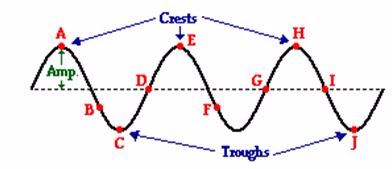1. A transverse
wave is a wave in which the particles of the medium are displaced in a
direction perpendicular to the direction of energy transport.
2. The crest
of a wave is the point on the medium that exhibits the maximum amount
of positive or upward displacement from the rest position.
3. The trough
of a wave is the point on the medium that exhibits the maximum amount
of negative or downward displacement from the rest position.
4. The amplitude
of a wave refers to the maximum amount of displacement of a particle on
the medium from its rest position. In a sense, the amplitude is the
distance from rest to crest. Similarly, the amplitude can be measured from the rest position to the trough position.
5. The wavelength
of a wave is simply the length of one complete wave cycle. If you were
to trace your finger across the wave in the diagram above, you would
notice that your finger repeats its path. A wave is a repeating pattern.
It repeats itself in a periodic and regular fashion over both time and
space. And the length of one such spatial repetition (known as a wave cycle)
is the wavelength. The wavelength can be measured as the distance from
crest to crest or from trough to trough. In fact, the wavelength of a
wave can be measured as the distance from a point on a wave to the
corresponding point on the next cycle of the wave.
6. A longitudinal
wave is a wave in which the particles of the medium are displaced in a
direction parallel to the direction of energy transport. A longitudinal
wave can be created in a slinky if the slinky is stretched out
horizontally and the end coil is vibrated back-and-forth in a horizontal
direction.
7. A compression is a point on a
medium through which a longitudinal wave is traveling that has the
maximum density. A region where the coils are spread apart, thus
maximizing the distance between coils, is known as a rarefaction.
8. A rarefaction
is a point on a medium through which a longitudinal wave is traveling
that has the minimum density. Points A, C and E on the diagram above
represent compressions and points B, D, and F represent rarefactions.
9. The frequency, (f)
of a wave refers to how often the particles of the medium vibrate when a
wave passes through the medium. Given this definition, it is reasonable
that the quantity frequency would have units of cycles/second, waves/second, vibrations/second, or something/second. Another unit for frequency is the Hertz
(abbreviated Hz) where 1 Hz is equivalent to 1 cycle/second. If a coil
of slinky makes 2 vibrational cycles in one second, then the frequency
is 2 Hz.
10. Period, (T) refers to the time that it takes to do something. When an event occurs repeatedly, then we say that the event is periodic and refer to the time for the event to repeat itself as the period. The period
of a wave is the time for a particle on a medium to make one complete
vibrational cycle. Period, being a time, is measured in units of time
such as seconds, hours, days or years. The period of orbit for the Earth
around the Sun is approximately 365 days; it takes 365 days for the
Earth to complete a cycle.
11. The speed of an
object refers to how fast an object is moving and is usually expressed
as the distance traveled per time of travel. In the case of a wave, the
speed is the distance traveled by a given point on the wave (such as a
crest) in a given interval of time.The SI unit of speed is m/s.
Wave equation
The wave equation shows the relationship between speed, wavelength and frequency of a wave.
The
diagrams below show several “snapshots” of the production of a wave
within a rope. The motion of the disturbance along the medium after
every one-fourth of a period is depicted. Observe that in the time it
takes from the first to the last snapshot, the hand has made one
complete back-and-forth motion.
A
period has elapsed. Observe that during this same amount of time, the
leading edge of the disturbance has moved a distance equal to one
complete wavelength. So in a time of one period, the wave has moved a
distance of one wavelength. Combining this information with the equation
for speed (speed = distance/time), it can be said that the speed of a
wave is also the wavelength/period.
Since
the period is the reciprocal of the frequency, the expression 1/f can
be substituted into the above equation for period. Rearranging the
equation yields a new equation of the form:
Speed = Wavelength • Frequency.The above equation is known as the wave equation. It states the mathematical relationship between the speed (v) of a wave and its wavelength (λ) and frequency (f). Using the symbols v, λ, and f, the equation can be rewritten asv = f • λ
Types of Waves
Identify types of waves
Previously
we classified the waves by considering the movement of the particles.
And now you’re going to find out the 2 types of waves according to the
media of propagation.
1. Mechanical Waves
2. Electromagnetic Waves
Mechanical Waves
Mechanical
waves are also called elastic waves as their propagation depends on the
elastic properties of the medium through which the waves pass
Mechanical
waves are divided into three categories: Transverse waves, longitudinal
waves, and surface waves. In transverse waves, the medium moves
perpendicular to the wave direction, and in longitudinal waves, the
medium moves parallel to the wave direction.
In
surface waves, both transverse and longitudinal waves mix in a single
medium. In very simple words, an electronic wave is that which travels
in a vacuum, and a mechanical wave is that which needs some medium for
traveling.
Examples of mechanical waves are Sound waves, Water waves , Ocean waves, Earth quake waves, Seismic waves
Electromagnetic Waves
Electromagnetic
waves are waves that have no medium to travel whereas mechanical waves
need a medium for its transmission. Examples of electromagnetic waves
The following are the differences between mechanical and electromagnetic waves.
• Electromagnetic waves travel in a vacuum whereas mechanical waves do not.
• The mechanical waves need a medium like water, air, or anything for it to travel.
• While an electromagnetic wave is called just a disturbance, a mechanical wave is considered a periodic disturbance.

TOPIC 2: ELECTROMAGNETISM

Magnetic Fields due to a Current-carrying Conductor
How Electric Current Produce a Magnetic Field
Explain how electric current produces a magnetic field
Electromagnetism
is the effect produced by the interaction of an electric current with a
magnetic field. The interaction can result in a force causing the
conductor carrying the current.
If,
on the other hand, a force is applied to a conductor (with no current)
in a magnetic field the resulting movement can result in a current being
noticed in the conductor.
When
the switch is closed an electric current flows through the conductor.
The electric current generates magnetic field around the conductor. This
will cause a deflection on the compass needle. The magnetic field
around a current-carrying conductor can be shown by means of magnetic
field lines.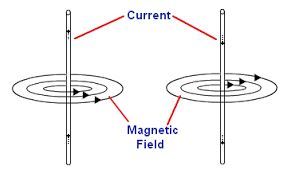The Pattern of the Magnetic Field Lines around a Straight Conductor
Identify the pattern of the magnetic field lines around a straight conductor
The
magnetic field pattern is usually given in a plan view. In the plan
view, the conductor is represented by a circle. A dot in circle shows
that the current is coming out of the plane. A cross the circle shows
that the current is moving into the plane.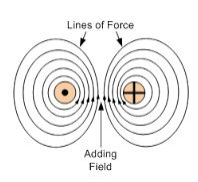The
strength of the magnetic field on the magnitude of the electric
current. The higher the current, the stronger the magnetic field, and
therefore the greater the deflection. The strength of the magnetic field
decreases as you move further from the conductor. There will be less
deflection as the compass is drawn from the current-carrying conductor.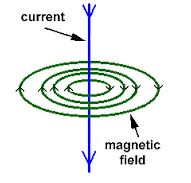The Direction of Magnetic Field around a Current-Carrying Conductor
Determine the direction of magnetic field around a current-carrying conductor
The direction of the field is determined by applying two rules, these are:
1. Right-hand Grip Rule
2. Maxwell’s cork screw rule
Right-hand Grip Rule
The
Right-hand Grip Rule can be applied to a straight conductor or a
solenoid-carrying an electric current. For a straight conductor, the
Right-hand Grip Rule can be stated as:
“Imagine
the wire carrying the current is gripped by the right hand with the
thumb pointing in the direction of the conventional current (from
positive to negative), the fingers will curl around the wire pointing in
the direction of the magnetic field.”For a solenoid, the Right-hand Grip Rule states that:
“When
you wrap your right hand around a solenoid with your fingers pointing
in the direction of convectional current, your thumb point in the
direction of the magnetic North pole.”
A solenoid is a long coil containing a large number of close turns of illustrated copper wire.
Maxwell’s –Right –hand screw rule states that:
“If
a right-hand screw advances in the direction of the current, then the
direction of rotation of the screw represents the direction of the
magnetic field due to the current.”The Presence and Direction of a Force on a Current carrying Conductor in a Magnetic Field
Determine the presence and direction of a force on a current carrying Conductor in a magnetic field
The
direction of the force on a current-carrying conductor in a magnetic
field can be determined using Fleming’s Left –Hand Rule.
Fleming’s Left –Hand Rule states that:
“If
you hold the index finger, the middle finger and the thumb of your left
hand mutually perpendicular to each other so that the index finger
points in the direction of the magnetic field and the middle finger
points in the direction of current in the conductor, then the thumb will
point in the direction of the force acting on the conductor.”The Direction of Force due to two Current carrying Conductors when the Current Flowing in the Same or Opposite Direction
Determine
the direction of force due to two current ’82air-carrying conductors
when the current flowing in the same or opposite direction
If
two current-carrying conductors are placed side by side close to one
another, the currents in the conductors will interact with the magnetic
fields produced by the two conductors. A force may result depending on
the direction of the two currents.
When
the currents are flowing in opposite directions, the conductors repel
one another. When the currents are flowing in the same direction, the
conductors attract one another, the conductors attract each other.
When
the currents flow in the same direction, the magnetic field between the
conductors cancel out, thus reducing the net field. However, on the
outside, the magnetic fields add up, thus increasing the net field.
Therefore, the magnetic field is weaker between the conductors that on
the outside. The resultant force pushes the conductor towards each
other.
When
the currents are in the opposite directions, the fields between the
conductors add up, while they cancel out on the outside. The field
between them is stronger than on the outside. The resultant force is
toward the outside of each conductor, hence repulsion.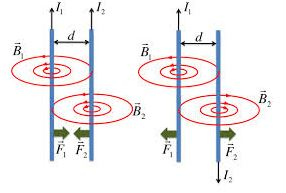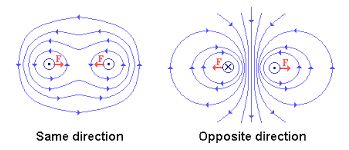ALL FORM FOUR TOPICS
FORM FOUR PHYSICS STUDY NOTES TOPIC 1: & TOPIC 2:

FORM FOUR PHYSICS STUDY NOTES TOPIC 3: & TOPIC 4:

FORM FOUR PHYSICS STUDY NOTES TOPIC 5: & TOPIC 6:

FORM FOUR PHYSICS STUDY NOTES TOPIC 7: GEOPHYSICS

#### 1 COMMENT

1. hongereni sana maktaba kwa msaada mlonipa sikuwa na vitabu lakin kupitia notes zenu nimesona nimefaulu